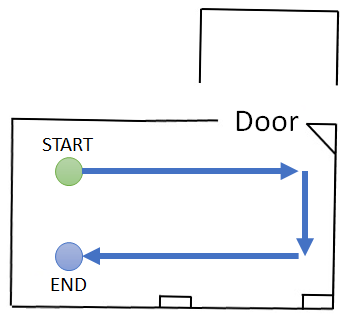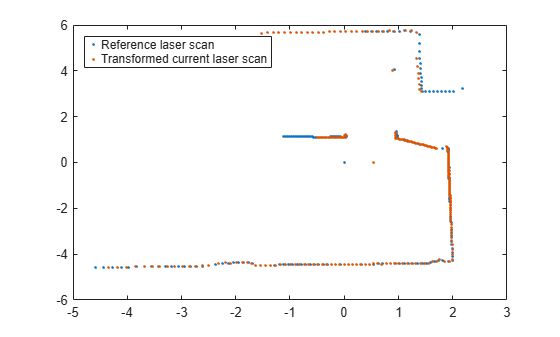Documentation

## Estimate Robot Pose with Scan Matching

This example demonstrates how to match two laser scans using the Normal Distributions Transform (NDT) algorithm . The goal of scan matching is to find the relative pose (or transform) between the two robot positions where the scans were taken. The scans can be aligned based on the shapes of their overlapping features.

To estimate this pose, NDT subdivides the laser scan into 2D cells and each cell is assigned a corresponding normal distribution. The distribution represents the probability of measuring a point in that cell. Once the probability density is calculated, an optimization method finds the relative pose between the current laser scan and the reference laser scan. To speed up the convergence of the method, an initial guess of the pose can be provided. Typically, robot odometry is used to supply the initial estimate.

If you apply scan matching to a sequence of scans, you can use it to recover a rough map of the environment that the robot traverses. Scan matching also plays a crucial role in other applications, such as position tracking and Simultaneous Localization and Mapping (SLAM).

### Load Laser Scan Data from File

`load lidarScans.mat`

The laser scan data was collected by a mobile robot in an indoor environment. An approximate floorplan of the area, along with the robot's path through the space, is shown in the following image.### Plot Two Laser Scans

Pick two laser scans to scan match from `lidarScans`. They should share common features by being close together in the sequence.

```referenceScan = lidarScans(180); currentScan = lidarScans(202);```

Display the two scans. Notice there are translational and rotational offsets, but some features still match.

```currScanCart = currentScan.Cartesian; refScanCart = referenceScan.Cartesian; figure plot(refScanCart(:,1),refScanCart(:,2),'k.'); hold on plot(currScanCart(:,1),currScanCart(:,2),'r.'); legend('Reference laser scan','Current laser scan','Location','NorthWest');```### Run Scan Matching Algorithm and Display Transformed Scan

Pass these two scans to the scan matching function. `matchScans` calculates the relative pose of the current scan with respect to the reference scan.

`transform = matchScans(currentScan,referenceScan)`
```transform = 1×3 0.5348 -0.0065 -0.0336 ```

To visually verify that the relative pose was calculated correctly, transform the current scan by the calculated pose using `transformScan`. This transformed laser scan can be used to visualize the result.

`transScan = transformScan(currentScan,transform);`

Display the reference scan alongside the transformed current laser scan. If the scan matching was successful, the two scans should be well-aligned.

```figure plot(refScanCart(:,1),refScanCart(:,2),'k.'); hold on transScanCart = transScan.Cartesian; plot(transScanCart(:,1),transScanCart(:,2),'r.'); legend('Reference laser scan','Transformed current laser scan','Location','NorthWest');```### Build Occupancy Grid Map Using Iterative Scan Matching

If you apply scan matching to a sequence of scans, you can use it to recover a rough map of the environment. Use the `occupancyMap` class to build a probabilistic occupancy grid map of the environment.

Create an occupancy grid object for a 15 meter by 15 meter area. Set the map's origin to be [-7.5 -7.5].

```map = occupancyMap(15,15,20); map.GridLocationInWorld = [-7.5 -7.5]```
```map = occupancyMap with properties: XWorldLimits: [-7.5000 7.5000] YWorldLimits: [-7.5000 7.5000] OccupiedThreshold: 0.6500 FreeThreshold: 0.2000 ProbabilitySaturation: [0.0010 0.9990] GridLocationInWorld: [-7.5000 -7.5000] DataType: 'double' DefaultValue: 0.5000 Resolution: 20 GridSize: [300 300] XLocalLimits: [0 15] YLocalLimits: [0 15] GridOriginInLocal: [0 0] LocalOriginInWorld: [-7.5000 -7.5000] ```

Pre-allocate an array to capture the absolute movement of the robot. Initialize the first pose as `[0 0 0]`. All other poses are relative to the first measured scan.

```numScans = numel(lidarScans); initialPose = [0 0 0]; poseList = zeros(numScans,3); poseList(1,:) = initialPose; transform = initialPose;```

Create a loop for processing the scans and mapping the area. The laser scans are processed in pairs. Define the first scan as reference scan and the second scan as current scan. The two scans are then passed to the scan matching algorithm and the relative pose between the two scans is computed. The `exampleHelperComposeTransform` function is used to calculate of the cumulative absolute robot pose. The scan data along with the absolute robot pose can then be passed into the `insertRay` function of the occupancy grid.

```% Loop through all the scans and calculate the relative poses between them for idx = 2:numScans % Process the data in pairs. referenceScan = lidarScans(idx-1); currentScan = lidarScans(idx); % Run scan matching. Note that the scan angles stay the same and do % not have to be recomputed. To increase accuracy, set the maximum % number of iterations to 500. Use the transform from the last % iteration as the initial estimate. [transform,stats] = matchScans(currentScan,referenceScan, ... 'MaxIterations',500,'InitialPose',transform); % The |Score| in the statistics structure is a good indication of the % quality of the scan match. if stats.Score / currentScan.Count < 1.0 disp(['Low scan match score for index ' num2str(idx) '. Score = ' num2str(stats.Score) '.']); end % Maintain the list of robot poses. absolutePose = exampleHelperComposeTransform(poseList(idx-1,:),transform); poseList(idx,:) = absolutePose; % Integrate the current laser scan into the probabilistic occupancy % grid. insertRay(map,absolutePose,currentScan,10); end```

### Visualize Map

Visualize the occupancy grid map populated with the laser scans.

```figure show(map); title('Occupancy grid map built using scan matching results');```Plot the absolute robot poses that were calculated by the scan matching algorithm. This shows the path that the robot took through the map of the environment.

```hold on plot(poseList(:,1),poseList(:,2),'bo','DisplayName','Estimated robot position'); legend('show','Location','NorthWest')```### References

 P. Biber, W. Strasser, "The normal distributions transform: A new approach to laser scan matching," in Proceedings of IEEE/RSJ International Conference on Intelligent Robots and Systems (IROS), 2003, pp. 2743-2748